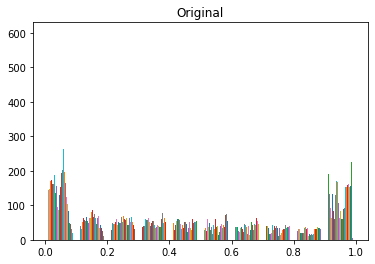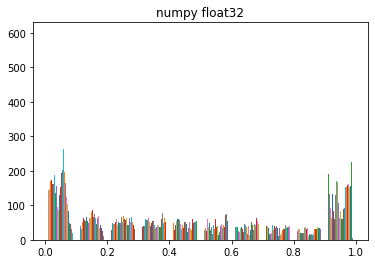# Histogram changes from numpy float32 to tensor float32

I’ve got some images I have pre-processed to do contrast adjustment and normalization. After the pre-processing, things look okay, but I’ve seen that the images returned from the dataloader (when I convert to tensor float32) don’t usually match what I saw before they were cast to tensors. The image is size 3xHxW, where the middle channel is actual raw image with the contrasting and the other two channels are random noise versions of that channel.

`skimage.exposure.equalize_adapthist`(image[, …]) Contrast Limited Adaptive Histogram Equalization (CLAHE).
which returns float64 output, then I normalize that and save it to a pickle file for loading later.

Code snippet to show histograms

``````img = pickle.load( open( fname, "rb" ) )
print('initial image type is ',type(img[1,0,0]))

plt.figure()
plt.hist(img[1,:,:])
plt.title('Original')

img2=img.astype(np.float32)
plt.figure()
plt.hist(img2[1,:,:])
plt.title('numpy float32')

img = torch.as_tensor(img, dtype=torch.float32)
plt.figure()
plt.hist(img[1,:,:])
plt.title('tensor float32')
``````

output:
initial image type is <class ‘numpy.float64’>I can guess that going from float64 to float32 might have to clip some numbers, if I had any in the range above float32, but I didn’t have any of those. Since the range between numbers might be less, could things be collapsing together onto the closest number in float32? If so, why does it only change for numpy float32 to a tensor and is not readily visible from numpy float64 to float32?

That’s an interesting issue indeed and I don’t think the numerical format creates the difference.
If you are using `plt.hist(img.numpy())` to pass the input as the expected numpy array to `hist`, you’ll get the same results, I guess internally `hist` might be treating the tensor differently than the numpy array.
Also, comparing each scalar between the tensor and numpy array yields a zero difference (if the numpy array is in `np.float32`).

EDIT: it seems transposing the tensor yields the same result: `plt.hist(img[1, :, :].t())`, but still unsure why that’s the case (probably the order of reading values as arrays are expected?).

1 Like

The difficulty is that pyplot.hist special cases “numpy.array” and treats tensors as sequences of sequences and “transposes it” (see the docstring). This is in contrast to other pyplot functions.
I’ve bumped into this more often than I’d like to admit, but never got around to tracking it down.

Best regards

Thomas

P.S.: The deed is done here: `lib/matplotlib/cbook/__init__.py` line 1372. If PyTorch provided to_numpy or PyPlot checked for `X.__array__()` instead, it would work. Maybe they could be inclined to use that.

2 Likes

Ah OK, this would also explain why `plt.hist(img2.tolist())` yields the same results as passing the tensor directly.

2 Likes